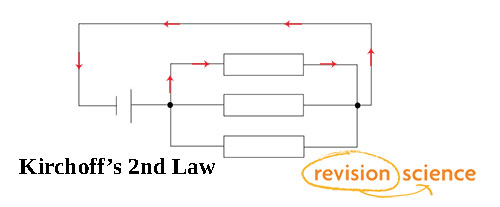## Title

Kirchoff's Laws

Kirchhoff's Laws

Kirchhoff’s Laws are Conservation Rules of a circuit.

Kirchoff's 1st Law (The Junction Rule) states that the total current that enters a junction is equal to the total current that leaves the junction.In the above diagram the current i1 is equal to the sum of currents i2, i3, and i4.

Here, the charge is conserved.

Kirchoff's 2nd Law (The Closed Loop Rule): Conservation of energy. The sum of voltages around a closed network is zero.

Can be also stated as 'the sum of emfs in a closed loop is equivilent to the sum of the potential drops in the loop'.An example of a closed loop

Here, the energy is conserved.

Below is a detailed video explanation of Kirshoff's Laws: